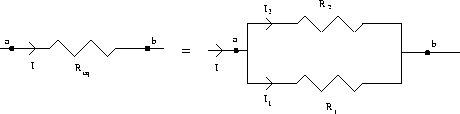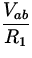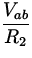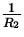## Resistors in Parallel

Suppose now in a section of a circuit we encounter a combination of two resistors as in Fig. 17.5.These resistors are said to be in parallel, and as before, it is possible to consider them as one single equivalent resistor R eq . To find this equivalent resistor, we exploit the fact from charge conservation that I = I1 + I2 . Using again Ohm's law (17.3), as well as the point that the potential difference across R1 and R2 is the same, we find this becomes=+=+(9)

This also is readily extended to the case of multiple resistors R1,R2,...,RN in parallel:=++...+(10)